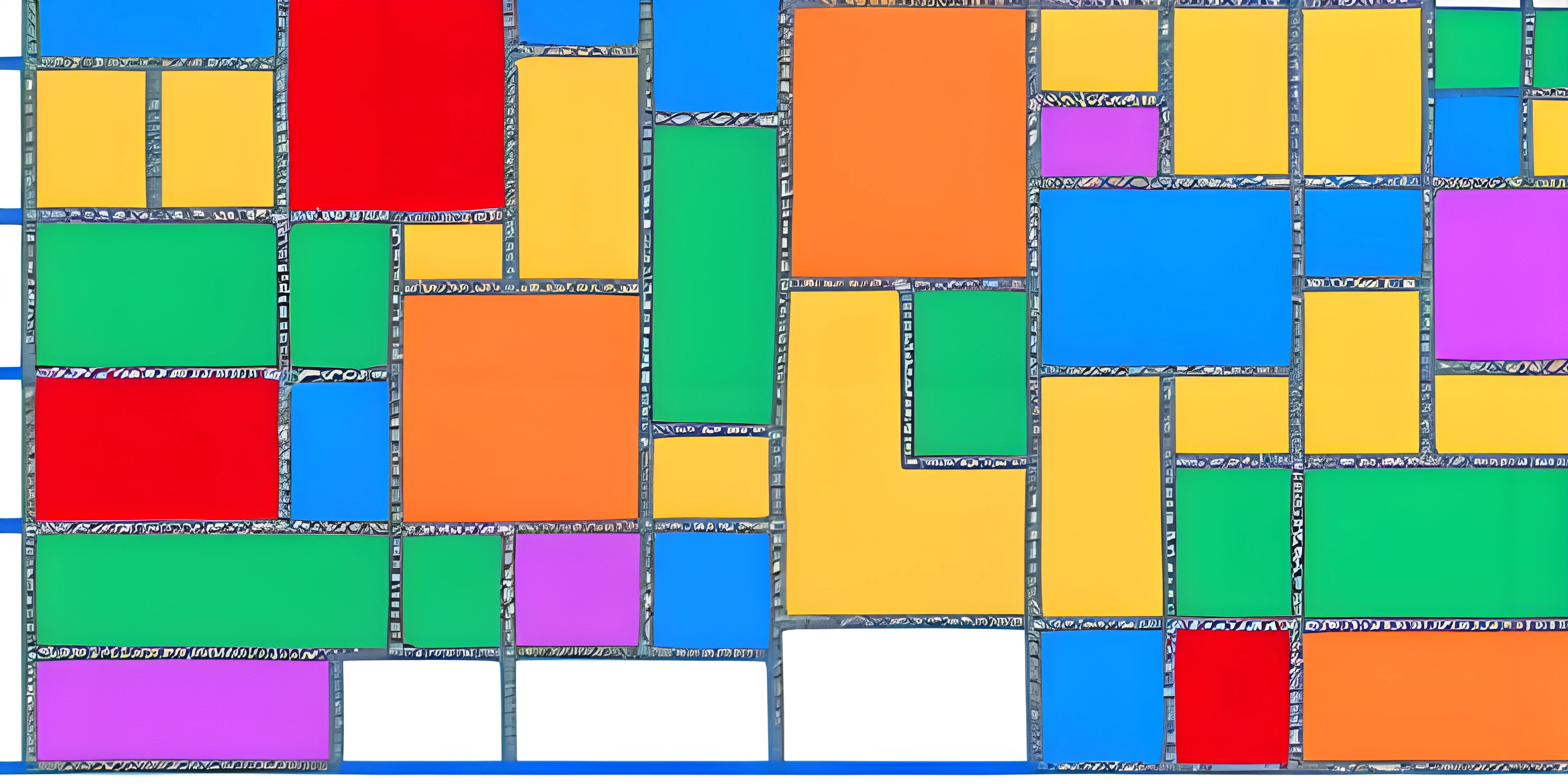# MATLAB PlottingNote: this page has been created with the use of AI. Please take caution, and note that the content of this page does not necessarily reflect the opinion of Cratecode.

Data visualization is an essential skill for any aspiring data scientist or engineer. With MATLAB, you can create stunning visualizations with just a few lines of code. In this article, we'll explore some of MATLAB's key plotting functions and learn how to create beautiful graphs and charts.

## Basic Plotting with MATLAB

MATLAB offers a wide variety of plotting functions that can be used to generate various types of data visualizations. Let's start by creating a simple line plot using the `plot()` function.

``````x = linspace(0, 2*pi, 100);
y = sin(x);
plot(x, y);``````

In this example, we first create an array `x` consisting of 100 evenly spaced values between 0 and 2π using the `linspace()` function. We then calculate the sine of each value in the `x` array and store the results in the `y` array. Finally, we use the `plot()` function to create a line plot of `y` against `x`.

MATLAB provides numerous options for customizing the appearance of your plots. You can change the color, linestyle, or marker style by specifying additional arguments in the `plot()` function.

``````x = linspace(0, 2*pi, 100);
y1 = sin(x);
y2 = cos(x);

plot(x, y1, 'r--', x, y2, 'b:');
legend('sin(x)', 'cos(x)');
xlabel('x-axis');
ylabel('y-axis');
title('Sine and Cosine Functions');``````

In this example, we plot two functions, sin(x) and cos(x), on the same graph. We customize the appearance of the lines using different colors (`r` for red and `b` for blue) and linestyles (`--` for dashed and `:` for dotted). Additionally, we add a legend (`legend()`), x-axis label (`xlabel()`), y-axis label (`ylabel()`), and a title (`title()`).

## Other Types of Plots

MATLAB offers many different types of plots, such as bar plots, pie charts, and histograms. Let's take a look at a few examples.

### Bar plots

``````categories = {'Category 1', 'Category 2', 'Category 3'};
values = [10, 20, 30];

bar(values);
set(gca, 'XTickLabel', categories);
ylabel('Value');
title('Bar Plot Example');``````

### Pie charts

``````categories = {'Category 1', 'Category 2', 'Category 3'};
values = [10, 20, 30];

pie(values, categories);
title('Pie Chart Example');``````

### Histograms

``````data = randn(1, 1000);

histogram(data, 25);
xlabel('Value');
ylabel('Frequency');
title('Histogram Example');``````

MATLAB's plotting capabilities are vast, and this article barely scratches the surface. We encourage you to explore the official MATLAB documentation to learn more about the different plotting functions available and how to customize them to your liking.

## FAQ

### How do I create a simple line plot in MATLAB?

You can create a simple line plot in MATLAB by using the `plot()` function. First, create two arrays for your x and y values, then call the `plot()` function with the x and y arrays as arguments.

### How can I customize the appearance of my plots in MATLAB?

You can customize the appearance of your plots in MATLAB by specifying additional arguments in the `plot()` function, such as color, linestyle, or marker style. You can also use functions like `legend()`, `xlabel()`, `ylabel()`, and `title()` to add labels and titles to your plot.

### What other types of plots can I create in MATLAB?

MATLAB offers a wide variety of plots, such as bar plots, pie charts, histograms, scatter plots, and more. You can use functions like `bar()`, `pie()`, `histogram()`, and `scatter()` to create these different types of plots.